# Test: Laplace Transform- 1

## 10 Questions MCQ Test Topicwise Question Bank for Electrical Engineering | Test: Laplace Transform- 1

Description
Attempt Test: Laplace Transform- 1 | 10 questions in 30 minutes | Mock test for Electronics and Communication Engineering (ECE) preparation | Free important questions MCQ to study Topicwise Question Bank for Electrical Engineering for Electronics and Communication Engineering (ECE) Exam | Download free PDF with solutions
QUESTION: 1

Solution:
QUESTION: 2

Solution:
QUESTION: 3

### Which of the following is the correct Laplace transform of the signal in the given figure?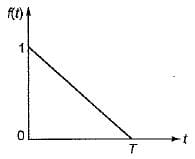Solution:
QUESTION: 4

Given: x(t) =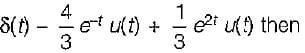find x(s) and its ROC:

Solution:
QUESTION: 5

Given: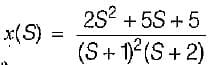and Re [S] >-1 then x (t):

Solution:
QUESTION: 6

If the input to LTI system is x(t) = e-3t u(t). Then the output is y(t) = [e-t - e-2t] u(t) the transfer function of the system is

Solution: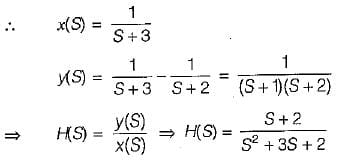QUESTION: 7

If,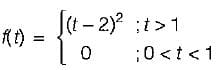then the value of L{tf(t)} is equal to

Solution:
QUESTION: 8

The transfer functions of the sytem is given by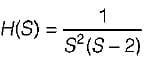The impulse response of the system is

Solution: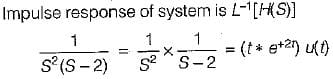QUESTION: 9

The Laplace transform of a continuous signal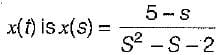If the Fourier transform of the signal exists, then x(t) is

Solution: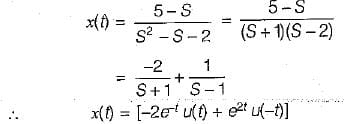QUESTION: 10

The laplace transform of i(t) is given by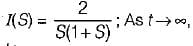the value of i(t) tends to

Solution: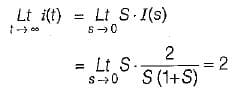Use Code STAYHOME200 and get INR 200 additional OFF Use Coupon Code